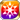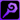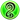# Twisted Time Sunk in Frigid Wastes

What in the world am I? That is the question I subconsciously ask of the jeweled orb in my hand. What I perceive in response, in the midst of all finite time, is a world of ice, somewhere between dream and reality. An indolent curse falls from my lips: "Humans are troublesome. May they all drown in the depths of their lust, just as this cold world has." Yet I am a student of Ambrosia, the Abstinent Witch, so I must not—will not—wish for such things. Still, as I sat there, I could not help but feel that such thoughts were a part of me, of who I am. (Nyx's thoughts, mid-trance)

## Stats

#### Simple stats

TypeLvl 1Lvl 30Lvl 40
MATK+10+24+30

LevelMATK
1+10
2+10
3+11
4+11
5+12
6+12
7+13
8+13
9+14
10+14
LevelMATK
11+15
12+15
13+16
14+16
15+17
16+17
17+18
18+18
19+19
20+19
LevelMATK
21+20
22+20
23+21
24+21
25+22
26+22
27+23
28+23
29+24
30+24
LevelMATK
31+25
32+25
33+26
34+26
35+27
36+27
37+28
38+28
39+29
40+30

## Group skills

### Lustburg's Galvanic Wall

#### Simple stats

TypeLvl 1Lvl 30Lvl 40
Thunder Res+1+4+6

LevelThunder Res
1+1
2+1
3+1
4+1
5+1
6+1
7+1
8+1
9+2
10+2
LevelThunder Res
11+2
12+2
13+2
14+2
15+2
16+2
17+3
18+3
19+3
20+3
LevelThunder Res
21+3
22+3
23+3
24+3
25+4
26+4
27+4
28+4
29+4
30+4
LevelThunder Res
31+4
32+4
33+5
34+5
35+5
36+5
37+5
38+5
39+5
40+6

#### Limit Break

TypeLB1LB2LB3LB4LB5
Thunder Res+1+1+2+3+4

### Lustburg's Galvanic Wall

#### Simple stats

TypeLvl 1Lvl 30Lvl 40
Thunder Res+1+4+6

LevelThunder Res
1+1
2+1
3+1
4+1
5+1
6+1
7+1
8+1
9+2
10+2
LevelThunder Res
11+2
12+2
13+2
14+2
15+2
16+2
17+3
18+3
19+3
20+3
LevelThunder Res
21+3
22+3
23+3
24+3
25+4
26+4
27+4
28+4
29+4
30+4
LevelThunder Res
31+4
32+4
33+5
34+5
35+5
36+5
37+5
38+5
39+5
40+6

#### Limit Break

TypeLB1LB2LB3LB4LB5
Thunder Res+1+1+2+3+4

### Twins' Ties That Bind

#### Simple stats

TypeLvl 1Lvl 30Lvl 40
MATK Debuff Res+13+33+40
Strong vs Fire+8+20+25

#### Detailed stats

LevelMATK Debuff ResStrong vs Fire
1+13+8
2+13+8
3+14+8
4+15+9
5+15+9
6+16+10
7+17+10
8+17+11
9+18+11
10+19+11
LevelMATK Debuff ResStrong vs Fire
11+19+12
12+20+12
13+21+13
14+22+13
15+22+14
16+23+14
17+24+14
18+24+15
19+25+15
20+26+16
LevelMATK Debuff ResStrong vs Fire
21+26+16
22+27+17
23+28+17
24+28+18
25+29+18
26+30+18
27+31+19
28+31+19
29+32+20
30+33+20
LevelMATK Debuff ResStrong vs Fire
31+33+21
32+34+21
33+35+21
34+35+22
35+36+22
36+37+23
37+37+23
38+38+24
39+39+24
40+40+25

#### Limit Break

TypeLB1LB2LB3LB4LB5
MATK Debuff Res+5+9+14+19+24
Strong vs Fire+3+6+9+12+15

TypeLB5
Area ATK+10

### Voidal Breeze

#### Simple stats

TypeLvl 1Lvl 30Lvl 40
HP+120+328+400
Hit Rate+3+8+10
Single Target ATK Res+2+6+8

#### Detailed stats

LevelHPHit RateSingle Target ATK Res
1+120+3+2
2+127+3+2
3+134+3+2
4+141+3+2
5+148+3+2
6+155+3+2
7+163+4+2
8+170+4+3
9+177+4+3
10+184+4+3
LevelHPHit RateSingle Target ATK Res
11+191+4+3
12+198+4+3
13+206+5+3
14+213+5+4
15+220+5+4
16+227+5+4
17+234+5+4
18+242+6+4
19+249+6+4
20+256+6+4
LevelHPHit RateSingle Target ATK Res
21+263+6+5
22+270+6+5
23+277+6+5
24+285+7+5
25+292+7+5
26+299+7+5
27+306+7+6
28+313+7+6
29+321+8+6
30+328+8+6
LevelHPHit RateSingle Target ATK Res
31+335+8+6
32+342+8+6
33+349+8+6
34+356+8+7
35+364+9+7
36+371+9+7
37+378+9+7
38+385+9+7
39+392+9+7
40+400+10+8

#### Limit Break

TypeLB1LB2LB3LB4LB5
HP+45+93+142+191+240
Hit Rate+1+2+3+4+6
Single Target ATK Res+1+2+3+4+5

TypeLB5
Wind+10

## Vision abilities

### Limit Reached Bonus

#### Ability base

• Slot:Support

Revealed after Limit Reached. Raises AGI after appearing on map.

#### Ability max

• Slot:Action

Raises AGI after appearing on map.

### [Time Warp] Backward Flow

#### Ability base

• Slot:Action
• DMG Type:Magical
• ATK Type:Magic
• Element:Wind

Wind Mag ATK on enemy units within area around self & greatly raises Power if target is inflicted with Delay [Area: Square (3), Height Range: 2]

Skill Effect
TypeMinMax
Attack120%143%
• Charges: 2
• Jewel Cost: 50
• Height: 2
• Select Scope: Square
• Scope: 1

#### Ability max

• Slot:Action
• DMG Type:Magical
• ATK Type:Magic
• Element:Wind

Wind Mag ATK on enemy units within area around self & greatly raises Power if target is inflicted with Delay [Area: Square (3), Height Range: 2]

Skill Effect
TypeMinMax
Attack221%221%
• Charges: 2
• Jewel Cost: 50
• Height: 2
• Select Scope: Square
• Scope: 1

### [Time Warp] Backward Flow

#### Ability base

• Slot:Action
• DMG Type:Magical
• ATK Type:Magic
• Element:Wind

Wind Mag ATK on enemy units within area around self & greatly raises Power if target is inflicted with Delay [Area: Square (3), Height Range: 2]

Skill Effect
TypeMinMax
Attack120%143%
• Charges: 2
• Jewel Cost: 50
• Height: 2
• Select Scope: Square
• Scope: 1

#### Ability max

• Slot:Action
• DMG Type:Magical
• ATK Type:Magic
• Element:Wind

Wind Mag ATK on enemy units within area around self & greatly raises Power if target is inflicted with Delay [Area: Square (3), Height Range: 2]

Skill Effect
TypeMinMax
Attack221%221%
• Charges: 2
• Jewel Cost: 50
• Height: 2
• Select Scope: Square
• Scope: 1